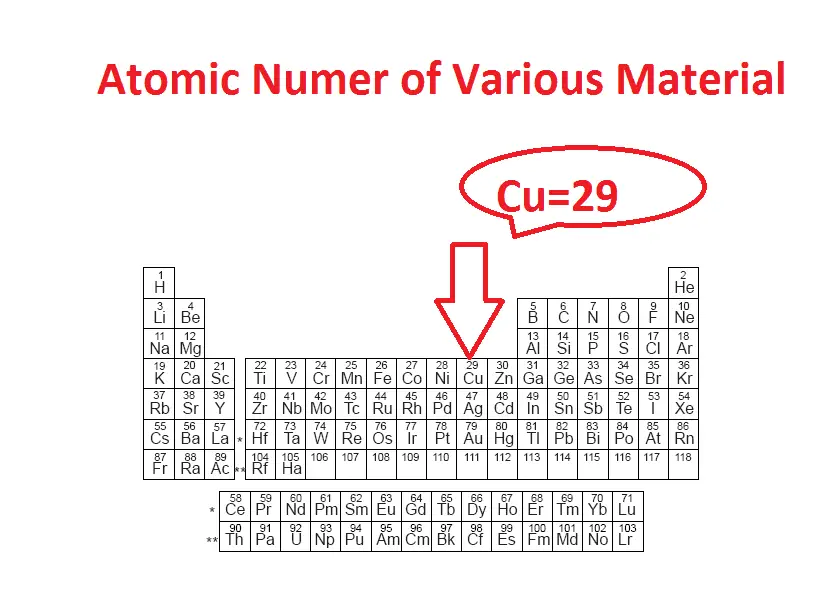# What is Free Electron and Basic Free Electron Concept?

## What is Free Electron and Basic Free Electron Concept?

An atom which contains three different charged particles and one is proton, electron, and neutron. In this proton and neutron will be inside of an atom’s nucleus and the electron revolves atom’s nucleus with specified orbit. Here electron contains the negative charge, the proton contains positive charge and the neutron do not have such charges. The number of proton and electron is always equal and opposite charges.

One electron charge is equal to 1.602 x 10^-19. The charge of the proton is equal and opposite to that of the electron, both charges are constant. Simply we can say The proton has a charge of +e, and the electron has a charge of −e.

If there are more electrons than protons in a piece of matter, it will have a negative charge, if there are less it will have a positive charge, and if there are equal numbers it will be neutral.

## Free electron:

The number of electrons in an orbit is given by 2n^2 where the small n is the number of orbits. The number of the electron per orbit varies. Let see

The electron in the first orbit is 2. The first orbit often denoted as k.

The electron in the second orbit is 8 and the second orbit is denoted by l.

The electron in the third orbit is 18 and the third orbit is denoted by m.

### How to identify the electron number?

Refer below-mentioned table. Which is the atomic number in the table? Here the numerical number indicates the total number of electron in a single atom.Example:

Copper atom has an atomic number of 29, which means single copper atom has 29 number of electrons.

Note: The Number of electrons is always equal to the number of protons.

Here the copper atom contained in the first orbit k= 2 electron

The second orbit’s electron = 8 electron

The third orbit’s electron = 18 electron

The fourth orbit’s electron = 1

Here the electron in the outmost orbit of an atom known as valence electron. This is the electron which determines the material chemical property such as conductor or insulator or semiconductor. Also it has less attraction with a nucleus so that a small external energy is applied to these atoms, the outmost electron gets detached or removed from the parent atom. Also they are free to move one atom to another atom. This electron is known as free electron which creates current flow in a conductor.

For the copper atom, a small heat energy at ordinary room temperature is enough to remove a vast number of the weekly held valance electron. Due to this, the copper has the larger number of free electron and it makes good conductor for electricity. Based on room temperature availability all the materials are classified into three categories

Learn More:   What is Resistor, Types, Series & Parallel Connection (Video Included)

1) Conductor

2) insulator

3) Semi-conductor